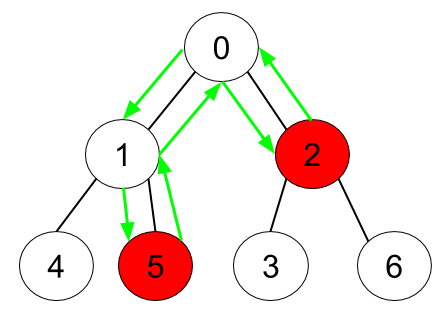# 1443. Minimum Time to Collect All Apples in a Tree

Medium
Given an undirected tree consisting of `n` vertices numbered from `0` to `n-1`, which has some apples in their vertices. You spend 1 second to walk over one edge of the tree. Return the minimum time in seconds you have to spend to collect all apples in the tree, starting at vertex 0 and coming back to this vertex.
The edges of the undirected tree are given in the array `edges`, where `edges[i] = [ai, bi]` means that exists an edge connecting the vertices `ai` and `bi`. Additionally, there is a boolean array `hasApple`, where `hasApple[i] = true` means that vertex `i` has an apple; otherwise, it does not have any apple.
Example 1:Input: n = 7, edges = [[0,1],[0,2],[1,4],[1,5],[2,3],[2,6]], hasApple = [false,false,true,false,true,true,false]
Output: 8
Explanation: The figure above represents the given tree where red vertices have an apple. One optimal path to collect all apples is shown by the green arrows.
Example 2:Input: n = 7, edges = [[0,1],[0,2],[1,4],[1,5],[2,3],[2,6]], hasApple = [false,false,true,false,false,true,false]
Output: 6
Explanation: The figure above represents the given tree where red vertices have an apple. One optimal path to collect all apples is shown by the green arrows.
Example 3:
Input: n = 7, edges = [[0,1],[0,2],[1,4],[1,5],[2,3],[2,6]], hasApple = [false,false,false,false,false,false,false]
Output: 0
Constraints:
• `1 <= n <= 10^5`
• `edges.length == n - 1`
• `edges[i].length == 2`
• `0 <= ai < bi <= n - 1`
• `fromi < toi`
• `hasApple.length == n`

### 解題

Runtime: 87 ms, faster than 100%
Memory Usage: 19.5 MB, less than 75%
func minTime(n int, edges [][]int, hasApple []bool) int {
g := make([][]int, n)
for i:=0; i<n; i++ {
g[i] = make([]int, 0)
}
for _, edge := range edges {
g[edge] = append(g[edge], edge)
g[edge] = append(g[edge], edge)
}
visited := make([]bool, n) // 紀錄哪些點有被走過
var helper func(int) int
helper = func(node int) int {
res := 0
visited[node] = true
for _, neight := range g[node] { // 遞迴 node 的鄰點，直到所有點都被 visited
if visited[neight] { continue }
res += helper(neight)
}
// res > 0 代表後面的點有蘋果要撿，這條路可以走
// 0 是起點，有蘋果不需要時間可以直接撿起來
if (res > 0 || hasApple[node]) && node != 0 {
res += 2
}
return res
}
return helper(0)
}